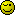# Is this sanitized enough?

Simple question:
Does

``````
preg_replace(\$_POST['bet'],'/[^0-9]*/','');

``````

sanitize a numerical input enough for use in a direct query() call?

For the OP’s particular code, it would appear that FILTER_SANITIZE_NUMBER_INT would be most appropriate, there’s also the option of providing a validating filter with a specific allowed range of values (e.g. accept only integers between 0 and 99999).

Also, it would generally be better to use the filter_input function for items like GET/POST input.

If you don’t like the (potential) verbosity of the filter functions, then something like ctype_digit would also check for only digits in a string.

Not quite; the OP wants to sanitize the input, not verify itFor numerical input the correct function to use to validate the field is is_numeric()

Okay. To sanitize numeric data rather than validating it you’d use:

filter_var(\$_POST[‘bet’], FILTER_SANITIZE_NUMBER_FLOAT);

Yeah… assuming i got the parameters in the right order, i mean… rolls eyes

By numerical i did mean integer in this case… though casting seems a lot simpler… which just makes me feel silly for not thinking of it.

Cerium: I normally would, but if i’m dropping everything but digits from it, the real_escape_string call is redundant, which is why i was asking.

I have a rule of thumb. Pass all user input through mysql_real_escape_string function or prepared statements.

You can however use ctype_* functions for your error handling

http://us3.php.net/manual/en/function.ctype-digit.php

If all you need is the numeric value of the variable use intval() function

http://us3.php.net/manual/en/function.intval.php

That would sanitise an integer input, but not all numerical values because they may contain decimal points.

That would be theoretical, however, because to sanitise integer inputs you simply use type casting!

``````\$SafeInteger = (int)\$_POST['integer'];
\$SafeFloat = (float)\$_POST['float'];
``````